# Acoustic signal processing with Python (2)

• I want to convert the sampling rate
• I want to read a 24-bit audio file with the wave module
• I want to estimate the group delay with scipy.signal.group_delay
• I want to frame using numpy's stride trick (I want to draw a spectrogram)
• I want to generate a TSP signal
• I want to visualize zeros and poles

## I want to convert the sampling rate

In order to convert the sampling rate, if the original sampling rate and the sampling rate you want to convert are integer ratios, you only need to use either up / down sampling, but in the case of rational ratios, use the least common multiple of each sampling rate. You need to upsample and then downsample to the desired sampling rate.

Here we consider converting the sampling rate from 48kHz to 44.1kHz. Sampling rate conversion can be realized with the following code. The target is the first 10 seconds of a 48kHz sound source.

``````#!/usr/bin/env python
# vim:fileencoding=utf-8

from fractions import Fraction

import numpy as np
import scipy as sp
import matplotlib.pyplot as plt

import scipy.signal as sg

import soundfile as sf

if __name__ == '__main__':
plt.close("all")

fs_target = 44100
cutoff_hz = 21000.0
n_lpf = 4096

sec = 10

wav_48kHz = wav[:fs_src * sec]

frac = Fraction(fs_target, fs_src)  # 44100 / 48000

up = frac.numerator  # 147
down = frac.denominator  # 160

# up sampling
wav_up = np.zeros(np.alen(wav_48kHz) * up)
wav_up[::up] = up * wav_48kHz
fs_up = fs_src * up

cutoff = cutoff_hz / (fs_up / 2.0)
lpf = sg.firwin(n_lpf, cutoff)

# filtering and down sampling
wav_down = sg.lfilter(lpf, , wav_up)[n_lpf // 2::down]

# write wave file
sf.write("down.wav", wav_down, fs_target)

# lowpass filter plot
w, h = sg.freqz(lpf, a=1, worN=1024)
f = fs_up * w / (2.0 * np.pi)
fig = plt.figure(1)
ax.semilogx(f, 20.0 * np.log10(np.abs(h)))
ax.axvline(fs_target, color="r")
ax.set_ylim([-80.0, 10.0])
ax.set_xlim([3000.0, fs_target + 5000.0])
ax.set_xlabel("frequency [Hz]")
ax.set_ylabel("power [dB]")

plt.show()
``````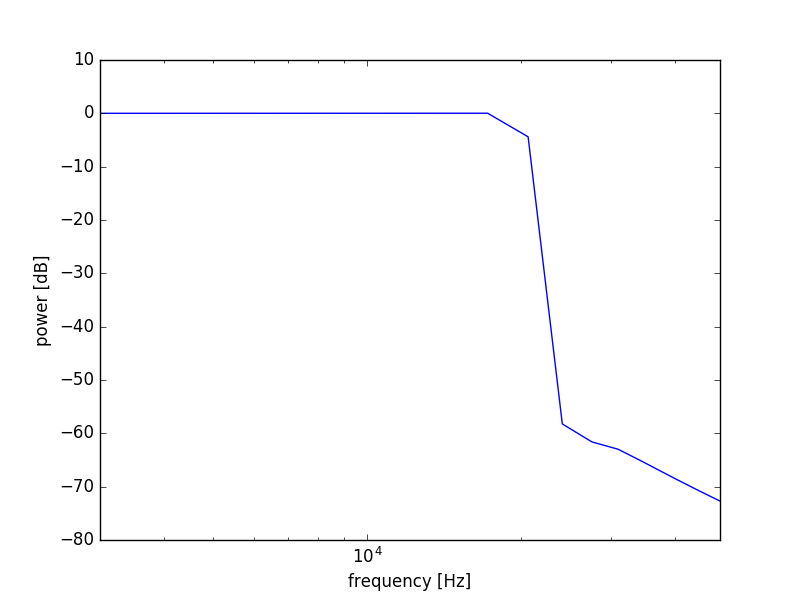Since 44100/48000 = 147/160, the original sound source is first upsampled to 147 times the score. Here, a buffer with a size of 147 times is created, and the original signal is substituted into the buffer at intervals of 147 points. As a result, the points that have not been assigned are zero-padded. Then design the LPF to include only frequencies below the Nyquist frequency of 44100Hz and use the lfilter to apply the LPF to limit the bandwidth. The point here is that the Nyquist frequency during upsampling is 48000/2 = 24000Hz, but after downsampling, 44100/2 = 22050Hz is the Nyquist frequency, so the band limiting filter applied during upsampling is 22050 or less. It is possible to use only one filter that becomes. After limiting the band, after correcting the delay due to the LPF, downsampling is performed at intervals of 160 points. As a result, sampling rate conversion was realized.

The actual audio waveform and spectrogram are as follows. The top two are after 44.1kHz conversion and the bottom two are before conversion.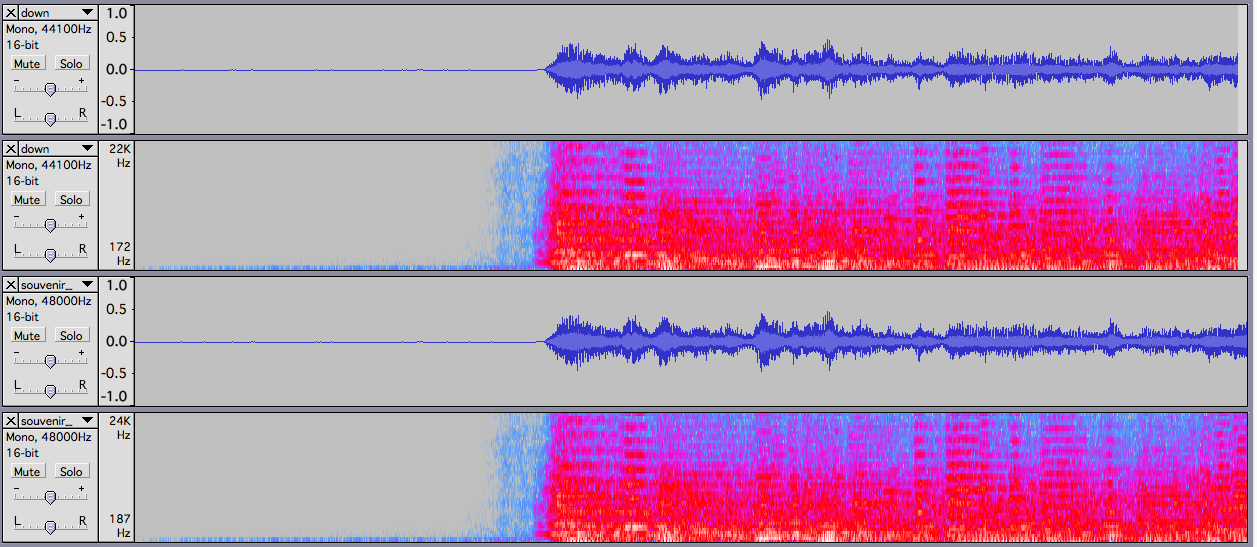## I want to read a 24-bit audio file with the wave module

If you want to read a 16-bit audio file with a standard wave module, you can read it with wave.open and read as much as you need with readframes and convert it to int16 with np.frombuffer, but in the case of a 24-bit sound source, you cannot specify 24bit with frombuffer. Therefore, you need to read it by yourself. Here, as shown in the code below, while reading 3 bytes at a time using the unpack of the struct module, the 24-bit sound source is read by packing 0s and unpacking as int32.

By the way, the sound source being read is e-onkyo sample sound source.

``````#!/usr/bin/env python
# vim:fileencoding=utf-8

import numpy as np
import matplotlib.pyplot as plt
import wave

from struct import unpack

if __name__ == '__main__':
plt.close("all")

fname = "../wav/souvenir.wav"
fp = wave.open(fname, "r")

nframe = fp.getnframes()
nchan = fp.getnchannels()
nbyte = fp.getsampwidth()
fs = fp.getframerate()

print("frame:{0}, "
"channel:{1}, "
"bytewidth:{2}, "
"fs:{3}".format(nframe, nchan, nbyte, fs))

fp.close()

#By packing 0 in the lowest bit and unpacking int
#Extract the value with a 24-bit value as a 32-bit int
#  (<i assumes little endian int value)
#unpack returns tupleI take the
unpacked_buf = [unpack("<i",
bytearray() + buf[nbyte * i:nbyte * (i + 1)])

#ndarray
ndarr_buf = np.array(unpacked_buf)

# -1.0〜1.Normalize to 0
float_buf = np.where(ndarr_buf > 0,
ndarr_buf / (2.0 ** 31 - 1),
ndarr_buf / (2.0 ** 31))

#Solve interleave(For stereo sound source)
wav_l = float_buf[::2]
wav_r = float_buf[1::2]
time = np.arange(np.alen(wav_l)) / fs

# plot
fig = plt.figure(1)

ax.plot(time, wav_l)
ax.set_xlabel("time [pt]")
ax.set_ylabel("amplitude")
ax.set_title("left channel")
ax.grid()

ax.plot(time, wav_r)
ax.set_xlabel("time [pt]")
ax.set_ylabel("amplitude")
ax.set_title("right channel")
ax.grid()

plt.show()
``````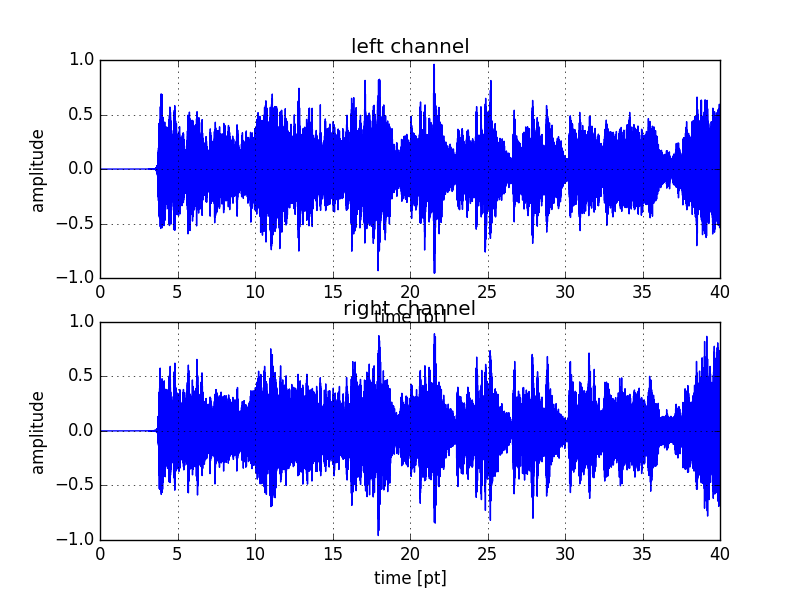## I want to estimate the group delay with scipy.signal.groupdelay

[This item] from last year's article (http://qiita.com/wrist/items/5759f894303e4364ebfd#%E7%BE%A4%E9%81%85%E5%BB%B6%E3%82%92%E8% A8% 88% E7% AE% 97% E3% 81% 97% E3% 81% 9F% E3% 81% 84) I didn't notice when I wrote it, but I didn't notice it, but the [group_delay method] for finding the group delay ( https://docs.scipy.org/doc/scipy-0.18.1/reference/generated/scipy.signal.group_delay.html#scipy.signal.group_delay) has been added since version 0.16.0 of scipy.signal. It was. This can be used to determine the group delay of a digital filter.

``````#!/usr/bin/env python
# vim:fileencoding=utf-8

import numpy as np
import matplotlib.pyplot as plt

import scipy.signal as sg

def allpass_filter(freq, r=0.9, fs=16000.0):
omega = 2.0 * np.pi * freq / fs

b = [r ** 2, - 2.0 * r * np.cos(omega), 1.0]
a = [1.0, -2.0 * r * np.cos(omega), r ** 2]

return (b, a)

if __name__ == '__main__':
plt.close("all")

fs = 16000.0
N = 1024

b, a = allpass_filter(1000.0)
w, h = sg.freqz(b, a, worN=N)

f = w * fs / (2.0 * np.pi)
_, gd = sg.group_delay((b, a), w=w)

fig = plt.figure(1)
ax.semilogx(f, np.abs(h))
ax.grid()
ax.set_xlabel("frequency [Hz]")
ax.set_ylabel("amplitude")
ax.set_xlim([10.0, fs / 2.0])

ax.semilogx(f, np.angle(h))
ax.grid()
ax.set_xlabel("frequency [Hz]")
ax.set_xlim([10.0, fs / 2.0])

ax.semilogx(f, gd)
ax.grid()
ax.set_xlabel("frequency [Hz]")
ax.set_ylabel("group delay [pt]")
ax.set_xlim([10.0, fs / 2.0])

plt.show()
``````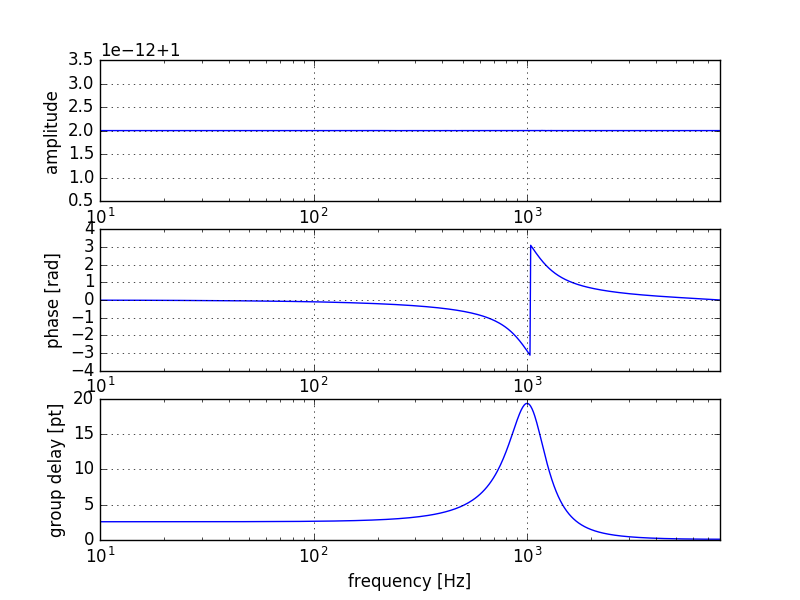## I want to do frame processing using numpy's stride trick (I want to draw a spectrogram)

Using the stride trick introduced in recipes 4.6 and 4.7 of IPython Data Science Cookbook, one buffer is divided into short frames. You can efficiently perform frame processing for repeated access without generating a copy of the frame. Using as_strided under numpy.lib.stride_tricks, you can write the method for calculating the spectrogram as follows.

``````#!/usr/bin/env python
#vim:fileencoding=utf-8

import numpy as np
import scipy as sp
import matplotlib.pyplot as plt
import matplotlib.cm as cm

import scipy.fftpack as fft
from numpy.lib.stride_tricks import as_strided

import soundfile as sf

def spectrogram(xs, nfft=1024, noverlap=2, win=np.hanning):

nsample = np.alen(xs)
nframe = int(nsample * (noverlap / float(nfft))) - noverlap
nbyte = xs.dtype.itemsize
shift = (nfft // noverlap)

xs_split = as_strided(xs, (nframe, nfft), (nbyte * shift, nbyte))
window = win(nfft)

Xs = []
print(nsample, nframe, nfft, shift, nbyte, nfft * nframe)
for frame in xs_split:
Xs.append(20.0 * np.log10(np.abs(fft.fft(window * frame, nfft))[:nfft//2 + 1]))

return np.array(Xs)

if __name__ == '__main__':
plt.close("all")

sec = 20

nfft = 1024

# plot
fig = plt.figure(1)
ax.grid()

ax.pcolor(np.arange(spec.shape),
np.arange(nfft//2 + 1) * (fs / nfft),
spec.T,
cmap=cm.jet)
ax.set_xlim([0, spec.shape])
ax.set_ylim([50, fs//2])
ax.set(yscale="log")

plt.show()
``````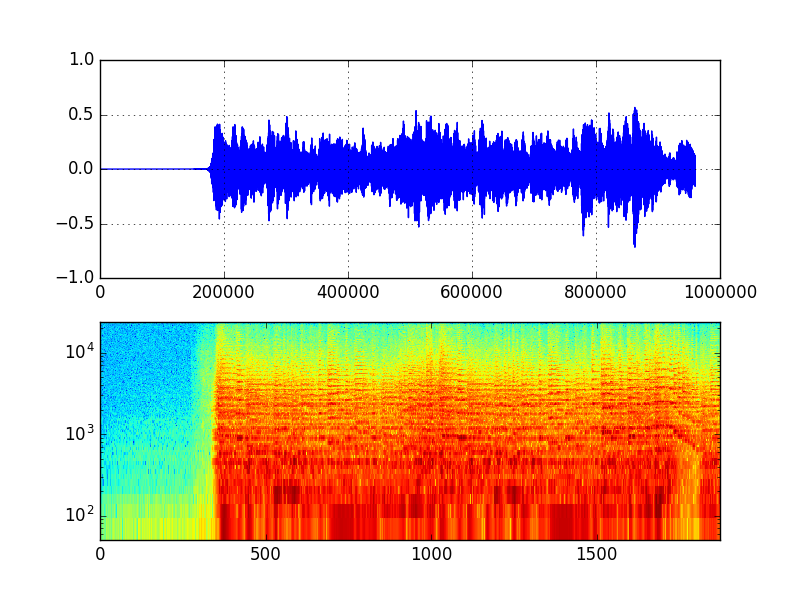stride is a value that represents the byte interval when accessing a contiguous area. If you change this, you will be able to control how many bytes you go ahead when you advance one row or column index.

as_strided is a method to access the buffer with this stride changed in a pseudo manner. The first argument is the access destination buffer, the second argument is the shape of the buffer created by changing the stride, and the third argument is the stride you want to change. If you access after changing the buffer of the first argument to the stride of the third argument in a pseudo manner, the operation will be such that an alias that can be accessed as the buffer indicated by the shape of the second argument is returned.

The format of this stride is (byte spacing when advancing rows, byte spacing when advancing columns). In the case of the above script, the original argument xs is a one-dimensional array and its stride is (8,), so if you advance the index by 1, it will advance by 8 bytes (dtype is float64). If this stride is changed to (512 * 8, 8) by, for example, as_stride, xs, which was originally a one-dimensional array, can be regarded as a two-dimensional array xs_split. If you repeatedly access xs_split with a for loop, each time you access it, it will go in the row direction of xs_split, that is, it will go 512 * 8 bytes ahead of xs, so you can access by shifting xs by 512pt. In this way, frame processing that would otherwise be processed by cutting out a one-dimensional array into multiple short-time frames can now be performed by stride tricks without causing copying at the time of cutting out. I will.

Note that this stride trick makes it easy to access outside the range of the internal buffer, so if you make a mistake, you will often access the undefined area and fall.

## I want to generate a TSP signal

When designing a Sweep Pulse (TSP signal) used for acoustic measurement, I think that it is often obtained by IFFTing what was designed in the frequency domain and returning it to the time domain. It can be generated with the following code. N_exp and m_exp, which contributes to the effective length, need adjustment.

``````#!/usr/bin/env python
# vim:fileencoding=utf-8

import numpy as np
import matplotlib.pyplot as plt

import scipy.fftpack as fft

import soundfile as sf

if __name__ == '__main__':
plt.close("all")

# parameters
N_exp = 16
m_exp = 2
nrepeat = 5
fs = 48000
gain = 100.0

N = 2 ** N_exp
m = N // (2 ** m_exp)  # (J=2m)
a = ((m * np.pi) * (2.0 / N) ** 2)

tsp_freqs = np.zeros(N, dtype=np.complex128)
tsp_freqs[:(N // 2) + 1] = np.exp(-1j * a * (np.arange((N // 2) + 1) ** 2))
tsp_freqs[(N // 2) + 1:] = np.conj(tsp_freqs[1:(N // 2)][::-1])

# ifft and real
tsp = np.real(fft.ifft(tsp_freqs, N))

# roll
tsp = gain * np.roll(tsp, (N // 2) - m)

# repeat
tsp_repeat = np.r_[np.tile(tsp, nrepeat), np.zeros(N)]

# write
sf.write("tsp.wav", tsp_repeat, fs)

fig = plt.figure(1)
ax.plot(tsp)
ax.plot(tsp_repeat)

plt.show()
``````

If you display the wav file generated by this with audacity, it will be as follows.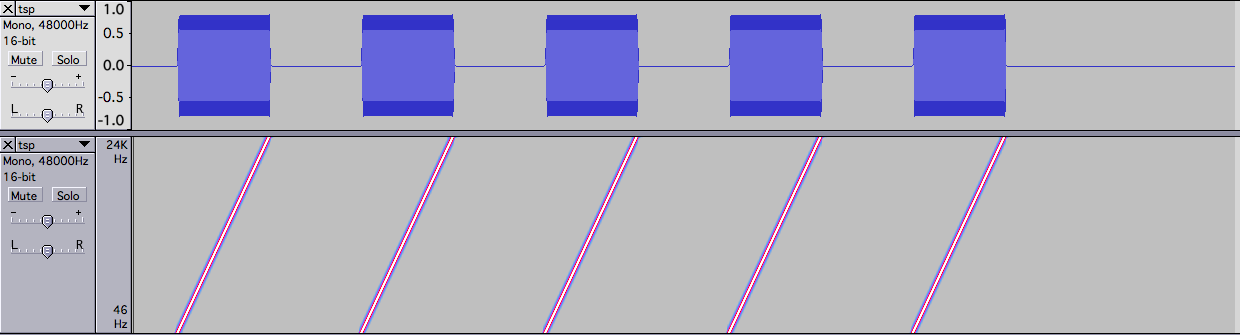The TSP signal is described in detail in Professor Kaneda's basic training materials on impulse response measurement below. http://www.asp.c.dendai.ac.jp/ASP/IRseminor2016.pdf

## I want to visualize zeros and poles

You can convert the time filter coefficient to zeros and poles with tf2zpk, but to visualize this, you can draw a circle using add_patch as shown below, and then plot without lines and with markers. .. I noticed after pasting the figure, but since it is an FIR filter, there is no pole, but please ignore it.

``````#!/usr/bin/env python
#vim:fileencoding=utf-8

import numpy as np
import scipy as sp
import matplotlib.pyplot as plt

import scipy.signal as sg

if __name__ == '__main__':
plt.close("all")

b = sg.firwin(11, 0.2, window="han")
z, p, k = sg.tf2zpk(b, )

fig = plt.figure(1, figsize=(8, 8))

ax.plot(np.real(z), np.imag(z), "o", mfc="white")
ax.plot(np.real(p), np.imag(p), "x", mfc="white")

ax.grid()
ax.set_xlim([-1.5, 1.5])
ax.set_ylim([-1.5, 1.5])

plt.show()
``````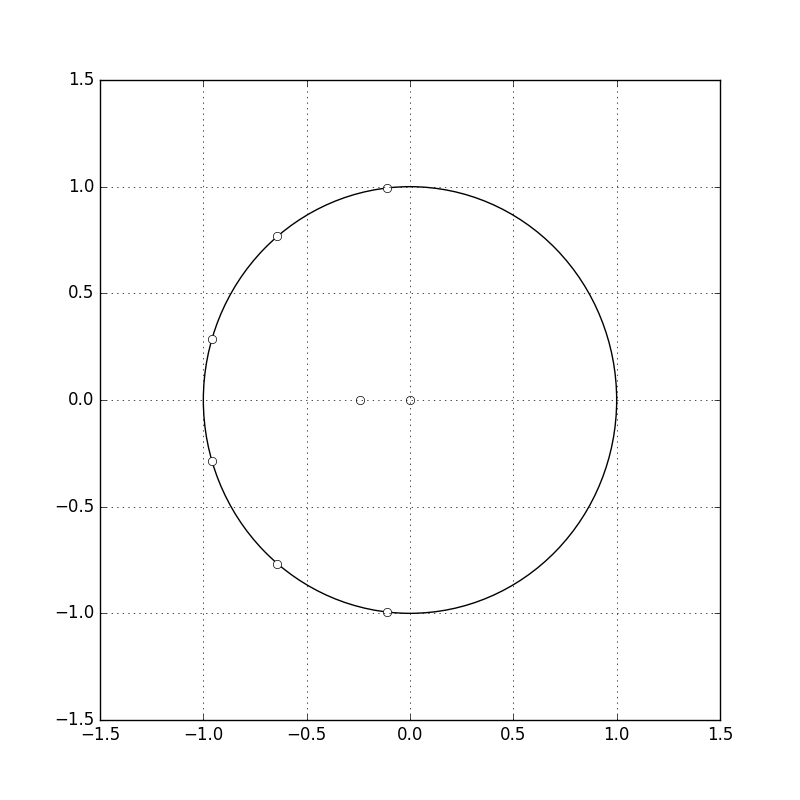## Other

I also wanted to write the following content, but I didn't have enough time, so I'd like to add it again or write it in another article.

• I want to estimate the impulse response from I / O signals
• Conversion of digital filter to SOS format
• I want to find the envelope of a signal using the Hilbert transform

Regarding the Hilbert transform, I wrote only the following explanation, so I will post it for the time being.

In order to find the envelope that smoothly traces the maximum amplitude of the signal, there is a method of multiplying the signal with the absolute value or square value by LPF, but also the method of finding it from the analysis signal obtained by the Hilbert transform. Has been done.

reference https://jp.mathworks.com/help/dsp/examples/envelope-detection.html